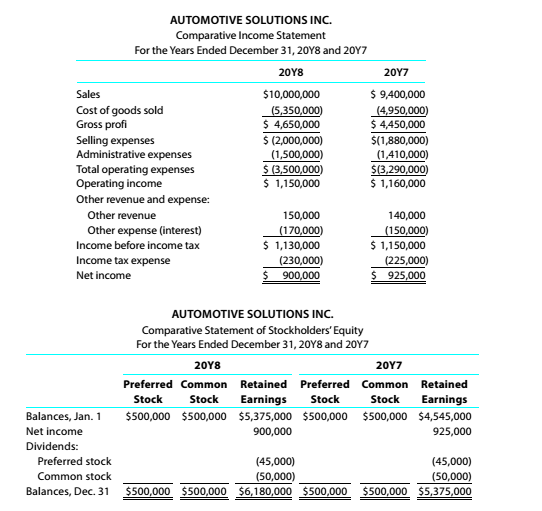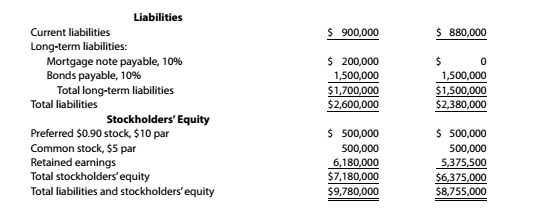Chapter 9, Problem 9.4.20P

Chapter
Section
Textbook Problem

Twenty metrics of liquidity, solvency, and profitabilityThe comparative financial statements of Automotive Solutions Inc. are as follows. The market price of Automotive Solutions Inc. common stock was $119.70 on December 31, 20Y8Instructions Dividend yield To determine Concept Introduction: Dividend Yield Ratio: Dividend Yield ratio is calculated as percentage by dividing the dividend per share by market price per share. The formula for the dividend yield ratio is as follows: Dividend Yield Ratio = Dividend per shareMarketprice per share To Calculate: The dividend yield. Explanation The dividend yield is calculated as follows:  20Y8 Common Dividend Paid (A)$ 50,000 Average Number of Common Shares outstanding (B)

Still sussing out bartleby?

Check out a sample textbook solution.

See a sample solution

The Solution to Your Study Problems

Bartleby provides explanations to thousands of textbook problems written by our experts, many with advanced degrees!

Get Started

The credit total of Factory Overhead represents actual factory overhead.

College Accounting, Chapters 1-27 (New in Accounting from Heintz and Parry)

Identify and briefly compare the two leading stock exchanges in the United States today.

Fundamentals of Financial Management, Concise Edition (MindTap Course List)

AFN EQUATION Refer to Problem 16-1 and assume that the company had 3 million in assets at the end of 2014. Howe...

Fundamentals of Financial Management, Concise Edition (with Thomson ONE - Business School Edition, 1 term (6 months) Printed Access Card) (MindTap Course List)

What is meant by business ethics?

Foundations of Business (MindTap Course List)